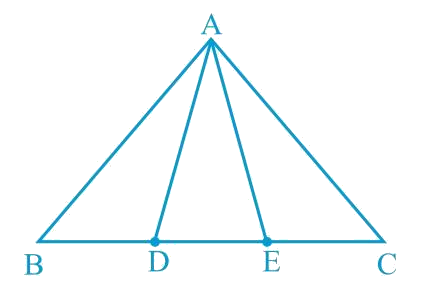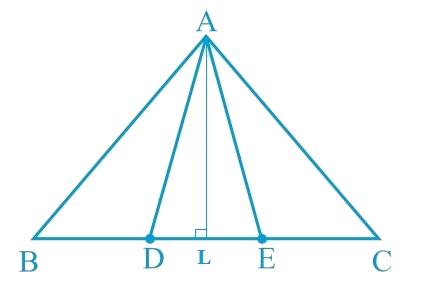# Ex.9.4 Q2 Areas of Parallelograms and Triangles Solution - NCERT Maths Class 9

Go back to  'Ex.9.4'

## Question

In the following figure, $$D$$ and $$E$$ are two points on $$BC$$ such that $$BD = DE = EC$$.
Show that:

$$ar (ABD) = ar (ADE) = ar (AEC)$$.

Video Solution
Areas Of Parallelograms And Triangles
Ex 9.4 | Question 2

## Text Solution

What is known?

$$D$$ and $$E$$ are two points on $$BC$$ such that  $$BD = DE = EC.$$

What is unknown?

How we can show that

$$\text{ar } \!\!( ABD ) = \!\text{ ar } \!\!( ADE ) = \text{ar } \!\! ( AEC ).$$

Reasoning:

First of all we can draw altitude which will help to find areas of all three triangles. As bases of all three triangles are equal so we can replace the value and we will find the required result.Can you now answer the question that you have left in the ’Introduction’ of this chapter, whether the field of Budhia has been actually divided into three parts of equal area?

[Remark: Note that by taking $$BD = DE = EC$$, the triangle $$ABC$$ is divided into three triangles $$ABD$$, $$ADE$$ and $$AEC$$ of equal areas. In the same way, by dividing $$BC$$ into n equal parts and joining the points of division so obtained to the opposite vertex of $$BC$$, you can divide $$\Delta \rm{ABC}$$  into n triangles of equal areas.]

Steps:

Let us draw a line segment $$AL ⊥ BC$$.

##We know that,

Area of a triangle $$= \frac{1}{2} \times$$ Base $$\times$$ Altitude

\begin{align} \text { Area }(\Delta {ADE})&=\frac{1}{2} \times {DE} \times {AL} \\ \text { Area }(\triangle {ABD})&=\frac{1}{2} \times {BD} \times {AL} \\ \text { Area }(\Delta {AEC})&=\frac{1}{2} \times {EC} \times {AL}\end{align}

It is given that

\begin{align}\frac{1}{2} \times DE \times AL &=\frac{1}{2} \times BD \times AL \\ &=\frac{1}{2} \times EC \times AL \\ \text { Area }(\Delta ADE)&=\text { Area }(\Delta ABD) \\ &=\text { Area }(\triangle AEC)\end{align}

It can be observed that Budhia has divided her field into  equal parts.

Video Solution
Areas Of Parallelograms And Triangles
Ex 9.4 | Question 2

Learn from the best math teachers and top your exams

• Live one on one classroom and doubt clearing
• Practice worksheets in and after class for conceptual clarity
• Personalized curriculum to keep up with school